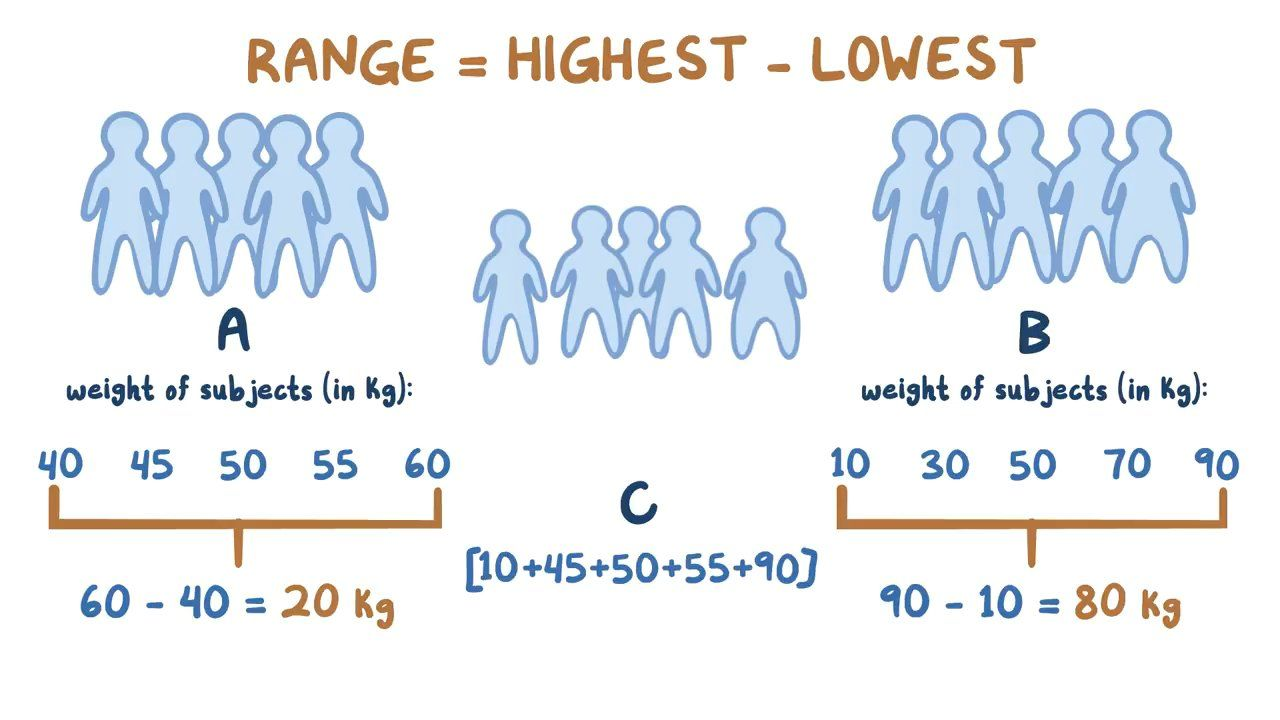[Image_Link]https://www.facebook.com/tr?id=824012811003867&ev=PageView&noscript=1 />

# Range, variance, and standard deviation

6,745views00:00 / 00:00

## Range, variance, and standard deviation

Biostatistics

### Introduction to biostatistics

Introduction to biostatistics

Types of data

Probability

### Statistical probability distributions

Mean, median, and mode

Range, variance, and standard deviation

Standard error of the mean (Central limit theorem)

Normal distribution and z-scores

### Parametric tests

Paired t-test

Two-sample t-test

Hypothesis testing: One-tailed and two-tailed tests

One-way ANOVA

Two-way ANOVA

Repeated measures ANOVA

Correlation

Methods of regression analysis

Linear regression

Logistic regression

### Non-parametric tests

Spearman's rank correlation coefficient

Mann-Whitney U test

Kappa coefficient

Chi-squared test

Fisher's exact test

Kaplan-Meier survival analysis

### Outcomes of statistical hypothesis testing

Type I and type II errors

### Assessments

Range, variance, and standard deviation

0 / 7 complete

0 / 2 complete

0 / 2 complete

of complete

of complete

### USMLE® Step 2 style questions USMLE

of complete

A study is conducted to measure the body weight of high school students. A total of 60 students are included in this study. The mean body weight is 78 kg and the variance is 30 kg2. Which of the following best describes the meaning of variance?

### Transcript

#### Content Reviewers

Rishi Desai, MD, MPHYifan Xiao, MD

#### Contributors

Evan Debevec-McKenney

To understand a set of data - having a single number like the mean or median gives us a one number summary, but understanding how the data is distributed is also very important - and that’s where the range, variance, and standard deviation can be helpful.

For example, let’s say we are looking at the weight of 10 people and we divided them into groups A and B.

```weight of group A(in kg) weight of group B(in kg) 40 45 50 55 60 10 30 50 70 90 .```

Mean = (40+45+50+55+60)/5= 50kg Mean = (10+30+50+70+90)/5= 50kg

Now, if you calculate the mean weight of group A and group B, you will find both of them have the same value of 50 kg, but the weights of individuals in group A are much more centered around the mean than in group B.

So let’s start by looking at the range, which is the difference between the highest and lowest value in a dataset.

In group A, we have (60-40)=20 kg, whereas in group B we have (90-10)=80 kg.

So far so good. But now, let’s say we have decided to include another group called group C.

Weight of Group C (in kg) 10 45 50 55 90. Mean = (10+45+50+55+90)/5= 50 kg Range 90-10 = 80kg

So even when we change two data points, group C still has the same mean and range as group B since it depends only on the highest and lowest values, thus it provides no information about how the rest of the data points are distributed.

In this situation, it’s clear that we need a better idea of how all of the values are distributed and to do that we can look at the variance.

To calculate the variance, which is written out as σ2, we take each data point (x), subtract it from the mean (x-bar), and then we square this value so we don’t end up with a negative number.

Next, we add up the squared values and divide that result with the total number of data points (n).

So, let’s use this formula to calculate the variance for group A, B, and C where all three had a mean of 50.

So, for group A, we get: (40-50)2+(45-50)2+(50-50)2+(55-50)2+(60-50)2/(5)=50 kg2

For group B, we get: (10-50)2+(30-50)2+(50-50)2+(70-50)2+(90-50)2 /(5) = 800 kg2

### Summary

Range, variance, and standard deviation are all measures of the spread or dispersion of a set of numerical data. The range is the difference between the highest and lowest values in the data set. Variance is a measure of how far each value in a set of data is from the mean value. Variance is calculated by taking the average of the squared differences of each value from the mean. The larger the variance, the more spread out the data is from the mean. Standard deviation is the square root of the variance and it measures the spread of data around the mean. The larger the standard deviation, the more spread out the data is from the mean.